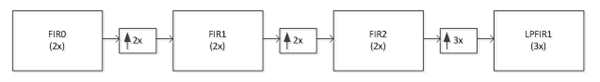# Digital signal processing in RF sampling DACs - part 1

In this blog post, I will discuss the important digital signal processing (DSP) blocks that create all of the magic in direct RF sampling DACs. These blocks include interpolation filters, mixers, numerically controlled oscillators (NCOs), summers and inverse sinc filters.

What makes RF sampling devices magical is that they combine digital interpolation and mixing to up-convert low-frequency signals to RF frequencies for transmitting signals wirelessly or over cable. Before RF sampling DACs, analog modulators were used for upconversion to RF with nonidealities (sideband images and local oscillator feedthrough).

So let’s begin with interpolation filters. Interpolation filters increase the input data rate so that the output rate is at least twice the desired RF output frequency. The higher output data rate increases the frequency separation between the desired RF signal and the inherent unwanted images, which makes filtering these images easier and less expensive. It also maximizes the RF output signal power because the desired signal will be in the first Nyquist zone, where sinc(x) attenuation is minimal.

Interpolation is usually accomplished in two stages: upsampling followed by digital low-pass filtering. The process of upsampling typically involves zero insertion or stuffing zeros between consecutive samples in time-domain. So to interpolate by N times, upsampling will insert N-1 zeros between consecutive samples in the time domain. The second stage of interpolation involves filtering out the images with a low-pass filter. The combined effect of zero stuffing and filtering in the frequency domain is that the foldover frequency will be shifted from Fdata/2 to (N/2)*Fdata, where Fdata is the input data rate. In RF sampling DACs, the interpolation factor (N) is high enough to place the foldover frequency in RF and consequently permit the DAC to directly generate RF signals without the need for an external analog modulator.

Direct RF sampling devices like the DAC38RF83 have various options for interpolation, from 6 to 24. These high-order interpolators are created by cascading several low-order interpolators (like two, three and five interpolators) as building blocks. Figure 1 shows how cascading several low-order interpolators creates an effective interpolation of 24.Figure 1: Implementing 24x interpolation through cascading

Obtaining the impulse response of the cascaded system shown in Figure 1 involves these steps:

1. Start with the impulse response of FIR0 (or the filter co-efficients of FIR0) and upsample by 2. An example MATLAB code is:

a. FIR0_2x = zeros(1,2*length(FIR0));

FIRO_2x(1:2:end) = FIR0;

2. Compute the convolution of upsampled data in step No. 1 with the filter coefficients of FIR1. An example MATLAB code is:

a. Interp_4x = conv(FIR0_2x,FIR1).

3. Upsample the time domain output samples of the FIR1 block by 2. An example MATLAB code is:

a. FIR1_2x = zeros(1,2*length(interp_4x));

b. FIR1_2x(1:2:end) = interp_4x;

4. Convolve the upsampled data in step 3 with the impulse response of the FIR2 block. An example MATLAB code is:

a. Interp_8x = conv(FIR1_2x,FIR2).

5. Upsample the time-domain output samples of the FIR2 block by 3. An example MATLAB code is:

a. FIR2_3x = zeros(1,3*length(interp_4x));

b. FIR2_3x(1:3:end) = interp_8x;

6. Convolve the upsampled data in step No. 5 with the impulse response of the LPFIR1 block to obtain the impulse response of the composite 24x interpolation filter. An example MATLAB code is:

a. Interp_24x = conv(FIR2_3x, LPFIR1).

Now that you know the impulse response of the interpolation filter, you can find the response of the interpolator to any time domain-sampled data input by simply computing the convolution of the sampled data and the impulse response. You can find the frequency response of the interpolators by computing the Fast Fourier Transform (FFT) of the time-domain impulse response. An example MATLAB code for the interp_24x impulse response computed above would be:

M = 1024 % number of FFT points

Interp_24x_fft = abs(fft(interp_24x),M))

Semilogy((1:M)/M,interp_24x_fft/max(interp_24x_fft))

Examining the magnitude frequency response will provide information such as the bandwidth, passband ripple and the stopband attenuation. For the interpolators implemented in the DAC38RF83, the bandwidth is approximately 0.4*Fdata, the passband ripple is less than 0.001dB and the stopband attenuation is greater than -80dBc.

In my next post, I will discuss another important DSP block in the RF sampling signal chain: mixers and NCOs.